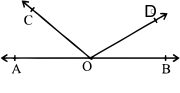6.7 MULTIPLE CHOICE QUESTIONS (M.C.Q’s)

1 ) A part of a line with two end points is called a _____________.
a ) ray b ) line segment c ) line d ) angle

2 ) A part of a line with one end point is called ____________.
a ) ray b ) line segment c ) line d ) angle

3 ) If three or more points lie on the same line then they are called ______ points.
a ) non collinear b ) collinear c ) intersecting d ) parallel

4 ) An angle that measures more than 900 but less than 1800 is called ______ angle.
a ) straight b ) acute c ) reflex d ) obtuse

5 ) An angle that measure more than 1800 but less than 3600 is called _______ angle.
a ) straight b ) acute c ) reflex d ) obtuse

6 ) If the sum of the two angles is _______ then it is called complementary angles.
a ) 360* b ) 90* c ) 180* d ) 270*

7 ) If a ray stands on a line then the sum of the two ___ angles so formed is 1800.
a ) adjacent b ) vertically opposite c ) interior opposite d ) linear

8 ) Name the following:
i ) 4 pairs of corresponding angles

ii ) 2 pairs of alternate interior angles

iii ) 2 pairs of alternate exterior angles
5 6
iv ) 2 pairs of interior angles on the same

side of the transversal

9 ) If ∠ ABD = ∠ DBC. Then the line BD is
called _____________
a ) transversal b ) angle bisector c ) segment d ) side

10 ) In this fig.
∠ AOC + ∠ COD + ∠ DOB = 180*What is the reason
a ) linear pair      b ) adjacent angle     c ) angles of a straight line is 1800
d ) supplementary angles

11 ) If the side of a triangle is produced the exterior angle so formed is equal to the sum of ________.
a ) opposite external angles b ) adjacent angles
c ) the two interior opposite angles d ) corresponding angles

1 ) line segment 2 ) ray 3 ) collinear 4 ) obtuse 5 ) reflex

6 ) 900 7 ) Adjacent

8 ) ( i ) ∠ 1 and ∠ 5, ∠ 2 and ∠ 6, ∠ 4 and ∠ 8, ∠ 3 and

∠ 7 ( ii ) ∠ 4 and ∠ 6, ∠ 3 and ∠ 5

( iii ) ∠ 1 and ∠ 7, ∠ 2 and ∠ 8 ( iv ) ∠ 4 and ∠ 5, ∠ 3 and ∠ 6
9 ) angle bisector 10 ) angles on a straight line is 1800

11 ) the two interior opposite angles